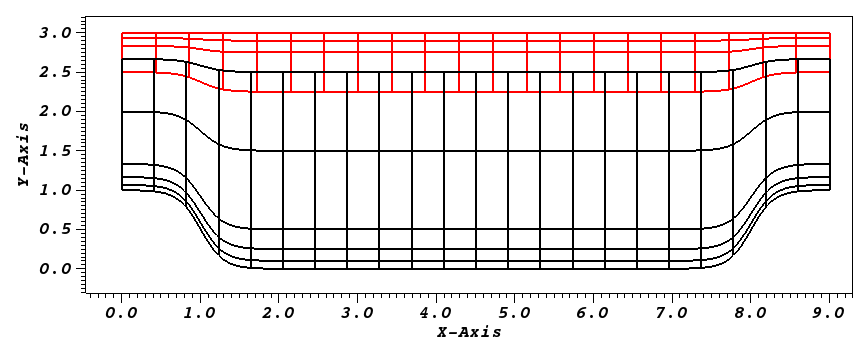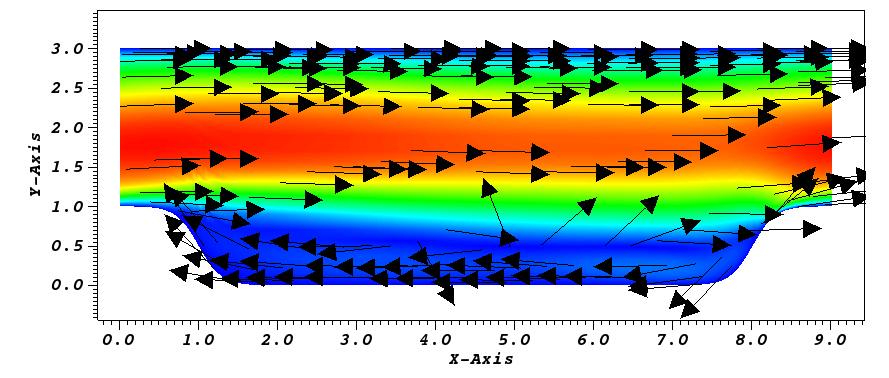# Overlapping Overset Grids¶

This tutorial will describe how to run a case with two overlapping meshes (NekNek) from scratch. We illustrate this by using the Periodic hill case shown above. If you are not familiar with Nek5000, we strongly recommend you begin with the Periodic hill first!

The three key steps to running a case with NekNek are:

1. Setting up the mesh with appropriate boundary conditions for the overlapping interface.
2. Specifying parameters to control stability and accuracy for the overlapping-Schwarz iterations.
3. Modifying userbc to read Dirichlet boundary data for the overlapping interfaces.

## Pre-processing¶

The pre-processing steps for a NekNek calculation differ from a mono-domain Nek5000 calculation only in terms of ensuring that the appropriate boundary condition int is specified at the overlapping interface. This boundary condition serves as the flag to let the solver know that Dirichlet data for these boundary conditions must come from interpolation of the data in the overlapping mesh.

## Mesh generation¶

In this tutorial, we generate two meshes to span the entire domain, an upper and a lower mesh. The lower mesh is generated by genbox with the following input file:

-2                     spatial dimension (will create box.re2)
1                      number of fields
#
#    comments: two dimensional periodic hill
#
#========================================================
#
Box                                       hillp
-22 5                                     Nelx  Nely
0.0 9.0 1.                                x0 x1 ratio
0.0 0.1 0.25 0.5 1.5 2.5                  y0 y1 ratio
P  ,P  ,W  ,int                           BC's:  (cbx0, cbx1, cby0, cby1)


and the upper mesh is generated by genbox with the following input file:

-2                     spatial dimension (will create box.re2)
1                      number of fields
#
#    comments: two dimensional periodic hill
#
#========================================================
#
Box                                       hillp
-21 3                                     Nelx  Nely
0.0 9.0 1.                                x0 x1 ratio
2.25 2.75 2.9 3.0     y0 y1 ratio
P  ,P  ,int,W                             BC's:  (cbx0, cbx1, cby0, cby1)


Note: the lower domain spans $$y \in [0,2.5]$$ and the upper domain spans $$y \in [2.25,3]$$. We also use int for the boundary surfaces that overlap the other domain.

Now the meshes can be generated by running genbox for each of these cases to obtain upper.re2 and lower.re2.

## usr file¶

As for the mono-domain periodic hill case, we start by copying the template to our case directory:

cp $HOME/Nek5000/core/zero.usr hillpnn.usr  ### Modify mesh and add forcing to the flow¶ As for the mono-domain periodic hill case, we modify the mesh in usrdat2 as: subroutine usrdat2 implicit none include 'SIZE' include 'TOTAL' integer ntot real sa,sb,sc,xx,argx,A1 ntot = nx1*ny1*nz1*nelt sa = 4.5 sb = 3.5 sc = 1./6 do i=1,ntot xx = xm1(i,1,1,1) argx = sb*(abs(xx-sa)-sb) A1 = sc + sc*tanh(argx) ym1(i,1,1,1) = ym1(i,1,1,1) + (3-ym1(i,1,1,1))*A1 enddo return endFig. 11 Modified box mesh graded Currently, applying a constant mass flux with param(54) and param(55) is not supported with overlapping overset grids. For this case, we drive the flow using a constant acceleration term in userf as:  subroutine userf(ix,iy,iz,eg) ! set acceleration term ! ! Note: this is an acceleration term, NOT a force! ! Thus, ffx will subsequently be multiplied by rho(x,t). ! implicit none integer ix,iy,iz,eg include 'SIZE' include 'TOTAL' include 'NEKUSE' integer e ! e = gllel(eg) ffx = 0.052 ffy = 0.0 ffz = 0.0 return end  ### Specify NekNek parameters¶ In usrdat, we specify the number of Schwarz-like iterations and extrapolation order for the boundary conditions of the overlapping interface. These parameters impact the stability and accuracy of the calculation. This is done as: subroutine usrdat() ! This routine to modify element vertices implicit none include 'SIZE' include 'TOTAL' include 'NEKNEK' ngeom = 5 ninter = 2 return end  Here we do 4 sub-iterations ($ngeom=5$) at each time-step between the two meshes, and the temporal extrapolation order ($ninter$) is 2. ### Initial & boundary conditions¶ The next step is to specify the initial conditions. This can be done in the subroutine useric as follows: subroutine useric(ix,iy,iz,ieg) implicit none integer ix,iy,iz,eg include 'SIZE' include 'TOTAL' include 'NEKUSE' ux = 1.0 uy = 0.0 uz = 0.0 temp = 0.0 return end  Next we modify userbc to read the boundary conditions, interpolated from overlapping domains, as follows:  subroutine userbc(ix,iy,iz,iside,eg) ! set up boundary conditions ! ! NOTE ::: This subroutine MAY NOT be called by every process ! implicit none integer ix,iy,iz,iside,eg include 'SIZE' include 'TOTAL' include 'NEKUSE' include 'NEKNEK' ie = gllel(eg) if (imask(ix,iy,iz,ie).eq.0) then ux=0.0 uy=0.0 uz=0.0 temp=0.0 else ux = valint(ix,iy,iz,ie,1) uy = valint(ix,iy,iz,ie,2) uz = valint(ix,iy,iz,ie,3) if (nfld_neknek.gt.3) temp = valint(ix,iy,iz,ie,ldim+2) end if return end  ## Control parameters¶ The control parameters for this case are the same as that for the mono-domain periodic hill case. Create two files called lower.par and upper.par, and type in each the following: # # nek parameter file # [GENERAL] stopAt = endTime endTime = 200 variableDT = yes targetCFL = 0.4 timeStepper = bdf2 writeControl = runTime writeInterval = 20 [PROBLEMTYPE] equation = incompNS [PRESSURE] residualTol = 1e-5 residualProj = yes [VELOCITY] residualTol = 1e-8 density = 1 viscosity = -100  ## SIZE file¶ The static memory layout of Nek5000 requires the user to set some solver parameters through a so called SIZE file. Typically it’s a good idea to start from our template. Copy the SIZE.template file from the core directory and rename it SIZE in the working directory: cp$HOME/Nek5000/core/SIZE.template SIZE


...

! BASIC
parameter (ldim=2)
parameter (lx1=8)
parameter (lxd=12)
parameter (lx2=lx1)

parameter (lelg=22*8)
parameter (lpmin=1)
parameter (lpmax=4)
parameter (ldimt=1)

! OPTIONAL
parameter (nsessmax=2)
...


For this tutorial we have set our polynomial order to be $$N=7$$ - this is defined in the SIZE file above as lx1=8 which indices that there are 8 points in each spatial dimension of every element. Additional details on the parameters in the SIZE file are given here.

## Compilation¶

With the hillpnn.usr, and SIZE files created, we are now ready to compile:

makenek hillpnn


If all works properly, upon compilation the executable nek5000 will be generated.

## Running the case¶

First we need to run our domain paritioning tool

genmap


On input specify lower as your casename and press enter to use the default tolerance. This step will produce lower.ma2 which needs to be generated only once. Next do the same for the upper mesh to generate upper.ma2.

Now you are all set, just run

neknekb lower upper 2 2


to launch an MPI jobs on your local machine using 4 ranks. The output will be redirected to logfile.

## Post-processing the results¶

Using Visit, the user can post-process the results.Fig. 12 Steady-State flow field visualized in Visit/Paraview. Vectors represent velocity. Colors represent velocity magnitude. Note, velocity vectors are equal size and not scaled by magnitude.